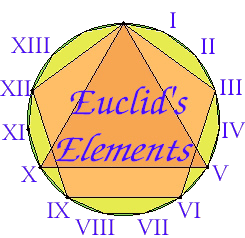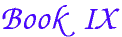Ok

En poursuivant votre navigation sur ce site, vous acceptez l'utilisation de cookies. Ces derniers assurent le bon fonctionnement de nos services. En savoir plus.

#### Propositions

Proposition 1.
If two similar plane numbers multiplied by one another make some number, then the product is square.
Proposition 2.
If two numbers multiplied by one another make a square number, then they are similar plane numbers.
Proposition 3.
If a cubic number multiplied by itself makes some number, then the product is a cube.
Proposition 4.
If a cubic number multiplied by a cubic number makes some number, then the product is a cube.
Proposition 5.
If a cubic number multiplied by any number makes a cubic number, then the multiplied number is also cubic.
Proposition 6.
If a number multiplied by itself makes a cubic number, then it itself is also cubic.
Proposition 7.
If a composite number multiplied by any number makes some number, then the product is solid.
Proposition 8.
If as many numbers as we please beginning from a unit are in continued proportion, then the third from the unit is square as are also those which successively leave out one, the fourth is cubic as are also all those which leave out two, and the seventh is at once cubic and square are also those which leave out five.
Proposition 9.
If as many numbers as we please beginning from a unit are in continued proportion, and the number after the unit is square, then all the rest are also square; and if the number after the unit is cubic, then all the rest are also cubic.
Proposition 10.
If as many numbers as we please beginning from a unit are in continued proportion, and the number after the unit is not square, then neither is any other square except the third from the unit and all those which leave out one; and, if the number after the unit is not cubic, then neither is any other cubic except the fourth from the unit and all those which leave out two.
Proposition 11.
If as many numbers as we please beginning from a unit are in continued proportion, then the less measures the greater according to some one of the numbers which appear among the proportional numbers.

Corollary. Whatever place the measuring number has, reckoned from the unit, the same place also has the number according to which it measures, reckoned from the number measured, in the direction of the number before it.

Proposition 12.
If as many numbers as we please beginning from a unit are in continued proportion, then by whatever prime numbers the last is measured, the next to the unit is also measured by the same.
Proposition 13.
If as many numbers as we please beginning from a unit are in continued proportion, and the number after the unit is prime, then the greatest is not measured by any except those which have a place among the proportional numbers.
Proposition 14.
If a number is the least that is measured by prime numbers, then it is not measured by any other prime number except those originally measuring it.
Proposition 15.
If three numbers in continued proportion are the least of those which have the same ratio with them, then the sum of any two is relatively prime to the remaining number.
Proposition 16.
If two numbers are relatively prime, then the second is not to any other number as the first is to the second.
Proposition 17.
If there are as many numbers as we please in continued proportion, and the extremes of them are relatively prime, then the last is not to any other number as the first is to the second.
Proposition 18.
Given two numbers, to investigate whether it is possible to find a third proportional to them.
Proposition 19.
Given three numbers, to investigate when it is possible to find a fourth proportional to them.
Proposition 20.
Prime numbers are more than any assigned multitude of prime numbers.
Proposition 21.
If as many even numbers as we please are added together, then the sum is even.
Proposition 22.
If as many odd numbers as we please are added together, and their multitude is even, then the sum is even.
Proposition 23.
If as many odd numbers as we please are added together, and their multitude is odd, then the sum is also odd.
Proposition 24.
If an even number is subtracted from an even number, then the remainder is even.
Proposition 25.
If an odd number is subtracted from an even number, then the remainder is odd.
Proposition 26.
If an odd number is subtracted from an odd number, then the remainder is even.
Proposition 27.
If an even number is subtracted from an odd number, then the remainder is odd.
Proposition 28.
If an odd number is multiplied by an even number, then the product is even.
Proposition 29.
If an odd number is multiplied by an odd number, then the product is odd.
Proposition 30.
If an odd number measures an even number, then it also measures half of it.
Proposition 31.
If an odd number is relatively prime to any number, then it is also relatively prime to double it.
Proposition 32.
Each of the numbers which are continually doubled beginning from a dyad is even-times even only.
Proposition 33.
If a number has its half odd, then it is even-times odd only.
Proposition 34.
If an [even] number neither is one of those which is continually doubled from a dyad, nor has its half odd, then it is both even-times even and even-times odd.
Proposition 35.
If as many numbers as we please are in continued proportion, and there is subtracted from the second and the last numbers equal to the first, then the excess of the second is to the first as the excess of the last is to the sum of all those before it.
Proposition 36.
If as many numbers as we please beginning from a unit are set out continuously in double proportion until the sum of all becomes prime, and if the sum multiplied into the last makes some number, then the product is perfect.

Next book: Book X

Previous: Book VIII

 Select from Book IX    Book IX intro    IX.1    IX.2    IX.3    IX.4    IX.5    IX.6    IX.7    IX.8    IX.9    IX.10    IX.11    IX.12    IX.13    IX.14    IX.15    IX.16    IX.17    IX.18    IX.19    IX.20    IX.21    IX.22    IX.23    IX.24    IX.25    IX.26    IX.27    IX.27    IX.28    IX.29    IX.30    IX.31    IX.32    IX.33    IX.34    IX.35    IX.36 Select book    Book I    Book II    Book III    Book IV    Book V    Book VI    Book VII    Book VIII    Book IX    Book X    Book XI    Book XII    Book XIII Select topic    Introduction    Table of Contents    Geometry applet    About the text    Euclid    Web references    A quick trip